# Tag: blackjack

About a year ago I did a series of posts on games associated to the Mathieu sporadic group $M_{12}$, starting with a post on Conway’s puzzle M(13), and, continuing with a discussion of mathematical blackjack. The idea at the time was to write a book for a general audience, as discussed at the start of the M(13)-post, ending with a series of new challenging mathematical games. I asked : “What kind of puzzles should we promote for mathematical thinking to have a fighting chance to survive in the near future?”

Now, Scientific American has (no doubt independently) taken up this lead. Their July 2008 issue features the article Rubik’s Cube Inspired Puzzles Demonstrate Math’s “Simple Groups” written by Igor Kriz and Paul Siegel.

By far the nicest thing about this article is that it comes with three online games based on the sporadic simple groups, the Mathieu groups $M_{12}$, $M_{24}$ and the Conway group $.0$.

the M(12) game

Scrambles to an arbitrary permutation in $M_{12}$ and need to use the two generators $INVERT=(1,12)(2,11)(3,10)(4,9)(5,8)(6,7)$ and $MERGE=(2,12,7,4,11,6,10,8,9,5,3)$ to return to starting position.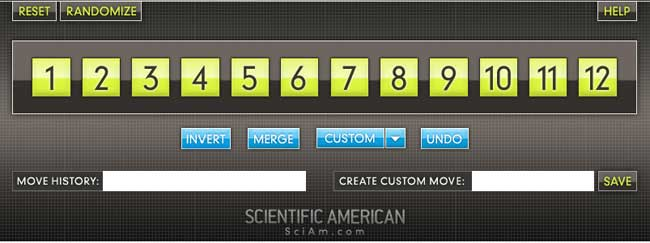Here is the help-screen :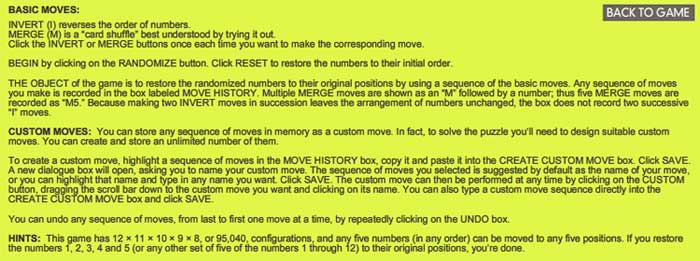They promise the solution by july 27th, but a few-line GAP-program cracks the puzzle instantly.

the M(24) game

Similar in nature, again using two generators of $M_{24}$. GAP-solution as before.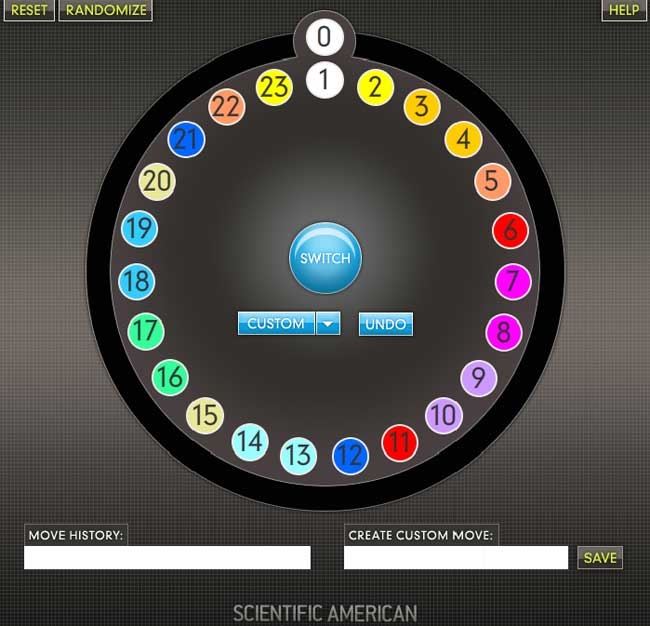This time, they offer this help-screen :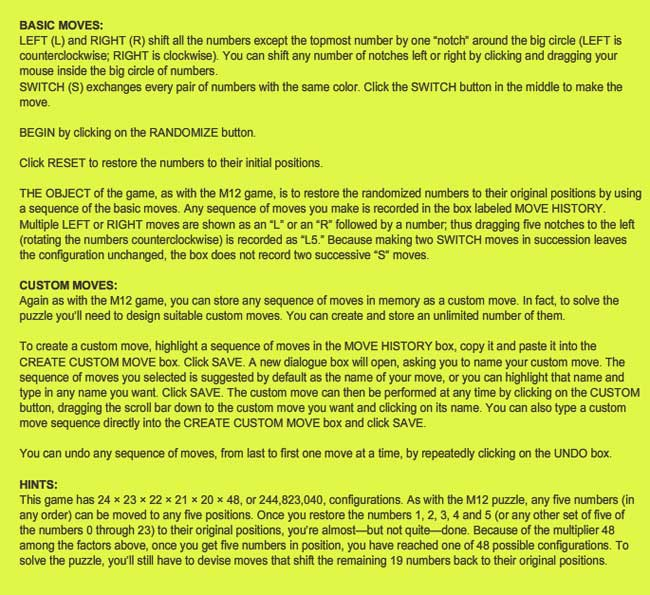the .0 game

Their most original game is based on Conway’s $.0$ (dotto) group. Unfortunately, they offer only a Windows-executable version, so I had to install Bootcamp and struggle a bit with taking screenshots on a MacBook to show you the game’s starting position :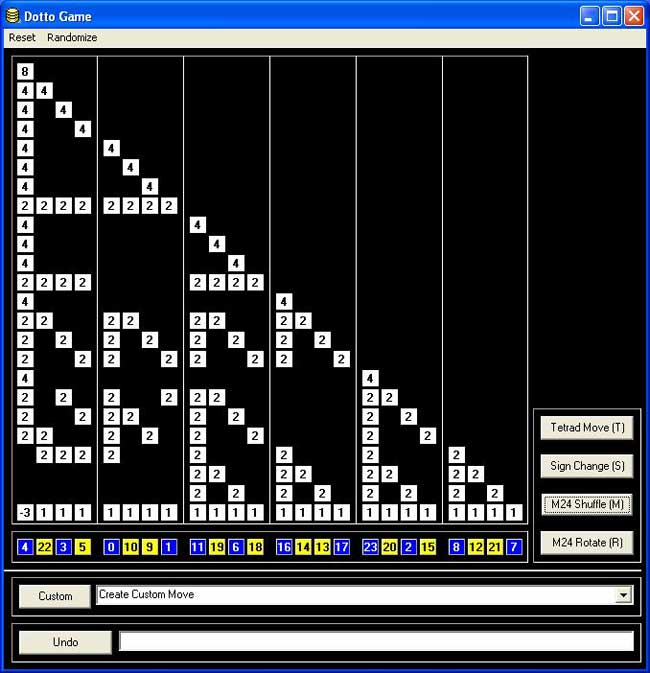Dotto:

Dotto, our final puzzle, represents the Conway group Co0, published in 1968 by mathematician John H. Conway of Princeton University. Co0 contains the sporadic simple group Co1 and has exactly twice as many members as Co1. Conway is too modest to name Co0 after himself, so he denotes the group “.0” (hence the pronunciation “dotto”).

In Dotto, there are four moves. This puzzle includes the M24 puzzle. Look at the yellow/blue row in the bottom. This is, in fact, M24, but the numbers are arranged in a row instead of a circle. The R move is the “circle rotation to the right”: the column above the number 0 stays put, but the column above the number 1 moves to the column over the number 2 etc. up to the column over the number 23, which moves to the column over the number 1. You may also click on a column number and then on another column number in the bottom row, and the “circle rotation” moving the first column to the second occurs. The M move is the switch, in each group of 4 columns separated by vertical lines (called tetrads) the “yellow” columns switch and the “blue” columns switch. The sign change move (S) changes signs of the first 8 columns (first two tetrads). The tetrad move (T) is the most complicated: Subtract in each row from each tetrad 1/2 times the sum of the numbers in that tetrad. Then in addition to that, reverse the signs of the columns in the first tetrad.

Strategy hints: Notice that the sum of squares of the numbers in each row doesn’t change. (This sum of squares is 64 in the first row, 32 in every other row.) If you manage to get an “8”in the first row, you have almost reduced the game to M24 except those signs. To have the original position, signs of all numbers on the diagonal must be +. Hint on signs: if the only thing wrong are signs on the diagonal, and only 8 signs are wrong, those 8 columns can be moved to the first 8 columns by using only the M24 moves (M,R).

MUBs (for Mutually Unbiased Bases) are quite popular at the moment. Kea is running a mini-series Mutual Unbias as is Carl Brannen. Further, the Perimeter Institute has a good website for its seminars where they offer streaming video (I like their MacromediaFlash format giving video and slides/blackboard shots simultaneously, in distinct windows) including a talk on MUBs (as well as an old talk by Wootters).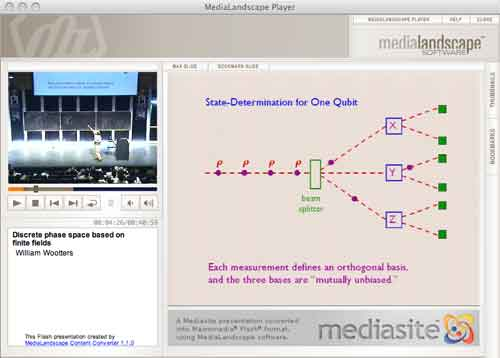So what are MUBs to mathematicians? Recall that a d-state quantum system is just the vectorspace $\mathbb{C}^d$ equipped with the usual Hermitian inproduct $\vec{v}.\vec{w} = \sum \overline{v_i} w_i$. An observable $E$ is a choice of orthonormal basis ${ \vec{e_i} }$ consisting of eigenvectors of the self-adjoint matrix $E$. $E$ together with another observable $F$ (with orthonormal basis ${ \vec{f_j} }$) are said to be mutally unbiased if the norms of all inproducts $\vec{f_j}.\vec{e_i}$ are equal to $1/\sqrt{d}$. This definition extends to a collection of pairwise mutually unbiased observables. In a d-state quantum system there can be at most d+1 mutually unbiased bases and such a collection of observables is then called a MUB of the system. Using properties of finite fields one has shown that MUBs exists whenever d is a prime-power. On the other hand, existence of a MUB for d=6 still seems to be open…

The King’s Problem (( actually a misnomer, it’s more the poor physicists’ problem… )) is the following : A physicist is trapped on an island ruled by a mean
king who promises to set her free if she can give him the answer to the following puzzle. The
physicist is asked to prepare a d−state quantum system in any state of her choosing and give it
to the king, who measures one of several mutually unbiased observables on it. Following this, the physicist is allowed to make a control measurement
on the system, as well as any other systems it may have been coupled to in the preparation
phase. The king then reveals which observable he measured and the physicist is required
to predict correctly all the eigenvalues he found.

The Solution to the King’s problem in prime power dimension by P. K. Aravind, say for $d=p^k$, consists in taking a system of k object qupits (when $p=2l+1$ one qupit is a spin l particle) which she will give to the King together with k ancilla qupits that she retains in her possession. These 2k qupits are diligently entangled and prepared is a well chosen state. The final step in finding a suitable state is the solution to a pure combinatorial problem :

She must use the numbers 1 to d to form $d^2$ ordered sets of d+1 numbers each, with repetitions of numbers within a set allowed, such that any two sets have exactly one identical number in the same place in both. Here’s an example of 16 such strings for d=4 :

11432, 12341, 13214, 14123, 21324, 22413, 23142, 24231, 31243, 32134, 33421, 34312, 41111, 42222, 43333, 44444

Here again, finite fields are used in the solution. When $d=p^k$, identify the elements of $\mathbb{F}_{p^k}$ with the numbers from 1 to d in some fixed way. Then, the $d^2$ of number-strings are found as follows : let $k_0,k_1 \in \mathbb{F}_{p^k}$ and take as the first 2 numbers the ones corresponding to these field-elements. The remaning d-2 numbers in the string are those corresponding to the field element $k_m$ (with $2 \leq m \leq d$) determined from $k_0,k_1$ by the equation

$k_m = l_{m} * k_0+k_1$

where $l_i$ is the field-element corresponding to the integer i ($l_1$ corresponds to the zero element). It is easy to see that these $d^2$ strings satisfy the conditions of the combinatorial problem. Indeed, any two of its digits determine $k_0,k_1$ (and hence the whole string) as it follows from
$k_m = l_m k_0 + k_1$ and $k_r = l_r k_0 + k_1$ that $k_0 = \frac{k_m-k_r}{l_m-l_r}$.

In the special case when d=3 (that is, one spin 1 particle is given to the King), we recover the tetracode : the nine codewords

0000, 0+++, 0—, +0+-, ++-0, +-0+, -0-+, -+0-, –+0

encode the strings (with +=1,-=2,0=3)

3333, 3111, 3222, 1312, 1123, 1231, 2321, 2132, 2213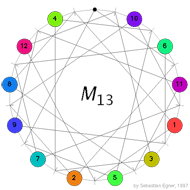Conway’s puzzle M(13) involves the 13 points and 13 lines of $\mathbb{P}^2(\mathbb{F}_3)$. On all but one point numbered counters are placed holding the numbers 1,…,12 and a move involves interchanging one counter and the ‘hole’ (the unique point having no counter) and interchanging the counters on the two other points of the line determined by the first two points. In the picture on the left, the lines are respresented by dashes around the circle in between two counters and the points lying on this line are those that connect to the dash either via a direct line or directly via the circle. In the first part we saw that the group of all reachable positions in Conway’s M(13) puzzle having the hole at the top positions contains the sporadic simple Mathieu group $M_{12}$ as a subgroup. To see the reverse inclusion we have to recall the definition of the ternary Golay code named in honour of the Swiss engineer Marcel Golay who discovered in 1949 the binary Golay code that we will encounter _later on_.

The ternary Golay code $\mathcal{C}_{12}$ is a six-dimenional subspace in $\mathbb{F}_3^{\oplus 12}$ and is spanned by its codewords of weight six (the Hamming distance of $\mathcal{C}_{12}$ whence it is a two-error correcting code). There are $264 = 2 \times 132$ weight six codewords and they can be obtained from the 132 hexads, we encountered before as the winning positions of Mathieu’s blackjack, by replacing the stars by signs + or – using the following rules. By a tet (from tetracodeword) we mean a 3×4 array having 4 +-signs indicating the row-positions of a tetracodeword. For example

$~\begin{array}{|c|ccc|} \hline & + & & \\ + & & + & \\ & & & + \\ \hline + & 0 & + & – \end{array}$ is the tet corresponding to the bottom-tetracodeword. $\begin{array}{|c|ccc|} \hline & + & & \\ & + & & \\ & + & & \\ \hline & & & \end{array}$ A col is an array having +-signs along one of the four columns. The signed hexads will now be the hexads that can be written as $\mathbb{F}_3$ vectors as (depending on the column-distributions of the stars in the hexad indicated between brackets)

$col-col~(3^20^2)\qquad \pm(col+tet)~(31^3) \qquad tet-tet~(2^30) \qquad \pm(col+col-tet)~(2^21^2)$

For example, the hexad on the right has column-distribution $2^30$ so its signed versions are of the form tet-tet. The two tetracodewords must have the same digit (-) at place four (so that they cancel and leave an empty column). It is then easy to determine these two tetracodewords giving the signed hexad (together with its negative, obtained by replacing the order of the two codewords)

$\begin{array}{|c|ccc|} \hline \ast & \ast & & \\ \ast & & \ast & \\ & \ast & \ast & \\ \hline – & + & 0 & – \end{array}$ signed as
$\begin{array}{|c|ccc|} \hline + & & & \\ & & & \\ & + & + & + \\ \hline 0 & – & – & – \end{array} – \begin{array}{|c|ccc|} \hline & + & & \\ + & & + & \\ & & & + \\ \hline + & 0 & + & – \end{array} = \begin{array}{|c|ccc|} \hline + & – & & \\ – & & – & \\ & + & + & \\ \hline – & + & 0 & – \end{array}$

and similarly for the other cases. As Conway&Sloane remark ‘This is one of many cases when the process is easier performed than described’.

We have an order two operation mapping a signed hexad to its negative and as these codewords span the Golay code, this determines an order two automorphism of $\mathcal{C}_{12}$. Further, forgetting about signs, we get the Steiner-system S(5,6,12) of hexads for which the automorphism group is $M_{12}$ hence the automorphism group op the ternary Golay code is $2.M_{12}$, the unique nonsplit central extension of $M_{12}$.

Right, but what is the connection between the Golay code and Conway’s M(13)-puzzle which is played with points and lines in the projective plane $\mathbb{P}^2(\mathbb{F}_3)$? There are 13 points $\mathcal{P}$ so let us consider a 13-dimensional vectorspace $X=\mathbb{F}_3^{\oplus 13}$ with basis $x_p~:~p \in \mathcal{P}$. That is a vector in X is of the form $\vec{v}=\sum_p v_px_p$ and consider the ‘usual’ scalar product $\vec{v}.\vec{w} = \sum_p v_pw_p$ on X. Next, we bring in the lines in $\mathbb{P}^2(\mathbb{F}_3)$.

For each of the 13 lines l consider the vector $\vec{l} = \sum_{p \in l} x_p$ with support the four points lying on l and let $\mathcal{C}$ be the subspace (code) of X spanned by the thirteen vectors $\vec{l}$. Vectors $\vec{c},\vec{d} \in \mathcal{C}$ satisfy the remarkable identity $\vec{c}.\vec{d} = (\sum_p c_p)(\sum_p d_p)$. Indeed, both sides are bilinear in $\vec{c},\vec{d}$ so it suffices to check teh identity for two line-vectors $\vec{l},\vec{m}$. The right hand side is then 4.4=16=1 mod 3 which equals the left hand side as two lines either intersect in one point or are equal (and hence have 4 points in common). The identity applied to $\vec{c}=\vec{d}$ gives us (note that the squares in $\mathbb{F}_3$ are {0,1}) information about the weight (that is, the number of non-zero digits) of codewords in $\mathcal{C}$

$wt(\vec{c})~mod(3) = \sum_p c_p^2 = (\sum_p c_p)^2 \in \{ 0,1 \}$

Let $\mathcal{C}’$ be the collection of $\vec{c} \in \mathcal{C}$ of weight zero (modulo 3) then one can verify that $\mathcal{C}’$ is the orthogonal complement of $\mathcal{C}$ with respect to the scalar product and that the dimension of $\mathcal{C}$ is seven whereas that of $\mathcal{C}’$ is six.
Now, let for a point p be $\mathcal{G}_p$ the restriction of

$\mathcal{C}_p = \{ c \in \mathcal{C}~|~c_p = – \sum_{q \in \mathcal{P}} c_q \}$

to the coordinates of $\mathcal{P} – \{ p \}$, then $\mathcal{G}_p$ is clearly a six dimensional code in a 12-dimensional space. A bit more work shows that $\mathcal{G}_p$ is a self-dual code with minimal weight greater or equal to six, whence it must be the ternary Golay code! Now we are nearly done. _Next time_ we will introduce a reversi-version of M(13) and use the above facts to deduce that the basic group of the Mathieu-groupoid indeed is the sporadic simple group $M_{12}$.

References

Robert L. Griess, “Twelve sporadic groups” chp. 7 ‘The ternary Golay code and $2.M_{12}$’

John H. Conway and N. J.A. Sloane, “Sphere packings, lattices and groups” chp 11 ‘The Golay codes and the Mathieu groups’

John H. Conway, Noam D. Elkies and Jeremy L. Martin, ‘The Mathieu group $M_{12}$ and its pseudogroup extension $M_{13}$’ arXiv:math.GR/0508630

If you only tune in now, you might want to have a look at the definition of Mathieu’s blackjack and the first part of the proof of the Conway-Ryba winning strategy involving the Steiner system S(5,6,12) and the Mathieu sporadic group $M_{12}$.

We’re trying to disprove the existence of misfits, that is, of non-hexad positions having a total value of at least 21 such that every move to a hexad would increase the total value. So far, we succeeded in showing that such a misfit must have the patern

$\begin{array}{|c|ccc|} \hline 6 & III & \ast & 9 \\ 5 & II & 7 & . \\ IV & I & 8 & . \\ \hline & & & \end{array}$

That is, a misfit must contain the 0-card (queen) and cannot contain the 10 or 11(jack) and must contain 3 of the four Romans. Now we will see that a misfit also contains precisely one of {5,6} (and consequently also exactly one card from {7,8,9}). To start, it is clear that it cannot contain BOTH 5 and 6 (then its total value can be at most 20). So we have to disprove that a misfit can miss {5,6} entirely (and so the two remaining cards (apart from the zero and the three Romans) must all belong to {7,8,9}).

Lets assume the misfit misses 5 and 6 and does not contain 9. Then, it must contain 4 (otherwise, its column-distribution would be (0,3,3,0) and it would be a hexad). There are just three such positions possible

$\begin{array}{|c|ccc|} \hline . & \ast & \ast & . \\ . & \ast & \ast & . \\ \ast & . & \ast & . \\ \hline – & – & ? & ? \end{array}$ $\begin{array}{|c|ccc|} \hline . & \ast & \ast & . \\ . & . & \ast & . \\ \ast & \ast & \ast & . \\ \hline – & + & ? & ? \end{array}$ $\begin{array}{|c|ccc|} \hline . & . & \ast & . \\ . & \ast & \ast & . \\ \ast & \ast & \ast & . \\ \hline – & 0 & ? & ? \end{array}$

Neither of these can be misfits though. In the first one, there is an 8->5 move to a hexad of smaller total value (in the second a 7->5 move and in the third a 7->6 move). Right, so the 9 card must belong to a misfit. Assume it does not contain the 4-card, then part of the misfit looks like (with either a 7- or an 8-card added)

$\begin{array}{|c|ccc|} \hline . & \ast & \ast & \ast \\ . & \ast & ? & . \\ . & \ast & ? & . \\ \hline & & & \end{array}$ contained in the unique hexad $\begin{array}{|c|ccc|} \hline \ast & \ast & \ast & \ast \\ . & \ast & & . \\ . & \ast & & . \\ \hline & & & \end{array}$

Either way the moves 7->6 or 8->6 decrease the total value, so it cannot be a misfit. Therefore, a misfit must contain both the 4- and 9-card. So it is of the form on the left below

$\begin{array}{|c|ccc|} \hline . & ? & \ast & \ast \\ . & ? & ? & . \\ \ast & ? & ? & . \\ \hline & & & \end{array}$ $\begin{array}{|c|ccc|} \hline . & . & \ast & . \\ . & \ast & \ast & \ast \\ \ast & \ast & . & . \\ \hline – & 0 & – & + \end{array}$ $\begin{array}{|c|ccc|} \hline . & . & \ast & \ast \\ . & \ast & \ast & . \\ \ast & \ast & . & . \\ \hline & & & \end{array}$

If this is a genuine misfit only the move 9->10 to a hexad is possible (the move 9->11 is not possible as all BUT ONE of {0,1,2,3,4} is contained in the misfit). Now, the only hexad containing 0,4,10 and 2 from {1,2,3} is in the middle, giving us what the misfit must look like before the move, on the right. Finally, this cannot be a misfit as the move 7->5 decreases the total value.

That is, we have proved the claim that a misfit must contain one of {5,6} and one of {7,8,9}. Right, now we can deliver the elegant finishing line of the Kahane-Ryba proof. A misfit must contain 0 and three among {1,2,3,4} (let us call the missing card s), one of $5+\epsilon$ with $0 \leq \epsilon \leq 1$ and one of $7+\delta$ with $0 \leq \delta \leq 2$. Then, the total value of the misfit is

$~(0+1+2+3+4-s)+(5+\epsilon)+(7+\delta)=21+(1+\delta+\epsilon-s)$

So, if this value is strictly greater than 21 (and we will see in a moment is has to be if it is at least 21) then we deduce that $s < 1 + \delta + \epsilon \leq 4$. Therefore $1+\delta+\epsilon$ belongs to the misfit. But then the move $1+\delta \epsilon \rightarrow s$ moves the misfit to a 6-tuple with total value 21 and hence (as we see in a moment) must be a hexad and hence this is a decreasing move! So, finally, there are no misfits!

Hence, from every non-hexad pile of total value at least 21 we have a legal move to a hexad. Because the other player cannot move from an hexad to another hexad we are done with our strategy provided we can show (a) that the total value of any hexad is at least 21 and (b) that ALL 6-piles of total value 21 are hexads. As there are only 132 hexads it is easy enough to have their sum-distribution. Here it is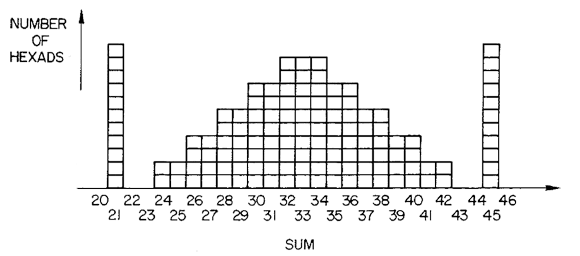That is, (a) is proved by inspection and we see that there are 11 hexads of sum 21 (the light hexads in Conway-speak) and there are only 11 ways to get 21 as a sum of 6 distinct numbers from {0,1,..,11} so (b) follows. Btw. the obvious symmetry of the sum-distribution is another consequence of the duality t->11-t discussed briefly at the end of part 2.

Clearly, I’d rather have conceptual proofs for all these facts and briefly tried my hand. Luckily I did spot the following phrase on page 326 of Conway-Sloane (discussing the above distribution) :

“It will not be easy to explain all the above observations. They are certainly connected with hyperbolic geometry and with the ‘hole’ structure of the Leech lattice.”

So, I’d better leave it at this…

References

Joseph Kahane and Alexander J. Ryba, “The hexad game

John H. Conway and N. J.A. Sloane, “Sphere packings, Lattices and Groups” chp. 11 ‘The Golay codes and the Mathieu groups’

(continued from part one). Take twelve cards and give them values 0,1,2,…,11 (for example, take the jack to have value 11 and the queen to have value 0). The hexads are 6-tuples of cards having the following properties. When we star their values by the scheme on the left below and write a 0 below a column if it has just one star at the first row or two stars on rows two and three (a + if the unique star is at the first row or two stars in the other columns, and a – if the unique star in on the second row or two stars in rows one and two) or a ? if the column has 3 or 0 stars, we get a tetracodeword where we are allowed to replace a ? by any digit. Moreover, we want that the stars are NOT distributed over the four columns such that all of the possible outcomes 0,1,2,3 appear once. For example, the card-pile { queen, 3, 4, 7, 9, jack } is an hexad as is indicated on the right below and has column-distributions (1,1,2,2).

$\begin{array}{|c|ccc|} \hline 6 & 3 & 0 & 9 \\ 5 & 2 & 7 & 10 \\ 4 & 1 & 8 & 11 \\ \hline & & & \end{array}$ $\begin{array}{|c|ccc|} \hline & \ast & \ast & \ast \\ & & \ast & \\ \ast & & & \ast \\ \hline – & 0 & – & + \end{array}$

The hexads form a Steiner-system S(5,6,12), meaning that every 5-pile of cards is part of a unique hexad. The permutations on these twelve cards, having the property that they send every hexad to another hexad, form the sporadic simple group $M_{12}$, the _Mathieu group_ of order 95040. For now, we assume these facts and deduce from them the Conway-Ryba winning strategy for Mathieu’s blackjack : the hexads are exactly the winning positions and from a non-hexad pile of total value at least 21 there is always a legal (that is, total value decreasing) move to an hexad by replacing one card in the pile by a card from the complement.

It seems that the first proof of this strategy consisted in calculating the Grundy values of all 905 legal positions in Mathieu’s blackjack. Later Joseph Kahane and Alex Ryba gave a more conceptual proof, that we will try to understand.

Take a non-hexad 6-pile such that the total value of its cards is at least 21, then removing any one of the six cards gives a 5-pile and is by the Steiner-property contained in a unique hexad. Hence we get 6 different hexads replacing one card from the non-hexad pile by a card not contained in it. We claim that at least one of these operations is a legal move, meaning that the total value of the cards decreases. Let us call a counterexample a misfit and record some of its properties until we can prove its non-existence.

A misfit is a non-hexad with total value at least 21 such that all 6 hexads, obtained from it by replacing one card by a card from its complement, have greater total value

A misfit must contain the queen-card. If not, we could get an hexad replacing one misfit-card (value > 0) by the queen (value zero) so this would be a legal move. Further, the misfit cannot contain the jack-card for otherwise replacing it by a lower-valued card to obtain an hexad is a legal move.

A misfit contains at least three cards from {queen,1,2,3,4}. If not, three of these cards are the replacements of misfit-cards to get an hexad, but then at least one of the replaced cards has a greater value than the replacement, giving a legal move to an hexad.

A misfit contains more than three cards from {queen=0, 1,2,3,4}. Assume there are precisely three $\{ c_1,c_2,c_3 \}$ from this set, then the complement of the misfit in the hexad {queen,1,2,3,4,jack} consists of three elements $\{ d_1,d_2,d_3 \}$ (a misfit cannot contain the jack). The two leftmost columns of the value-scheme (left above) form the hexad {1,2,3,4,5,6} and because the Mathieu group acts 5-transitively there is an element of $M_{12}$ taking $\{ 0,1,2,3,4,11 \} \rightarrow \{ 1,2,3,4,5,6 \}$ and we may even assume that it takes $\{ c_1,c_2,c_3 \} \rightarrow \{ 4,5,6 \}$. But then, in the new value-scheme (determined by that $M_{12}$-element) the two leftmost columns of the misfit look like

$\begin{array}{|c|ccc|} \hline \ast & . & ? & ? \\ \ast & . & ? & ? \\ \ast & . & ? & ? \\ \hline ? & ? & & \end{array}$

and the column-distribution of the misfit must be either (3,0,2,1) or (3,0,1,2) (it cannot be (3,0,3,0) or (3,0,0,3) otherwise the (image of the) misfit would be an hexad). Let {i,j} be the two misfit-values in the 2-starred column. Replacing either of them to get an hexad must have the replacement lying in the second column (in order to get a valid column distribution (3,1,1,1)). Now, the second column consists of two small values (from {0,1,2,3,4}) and the large jack-value (11). So, at least one of {i,j} is replaced by a smaller valued card to get an hexad, which cannot happen by the misfit-property.

Now, if the misfit shares four cards with {queen,1,2,3,4} then it cannot contain the 10-card. Otherwise, the replacement to get an hexad of the 10-card must be the 11-card (by the misfit-property) but then there would be another hexads containing five cards from {queen,0,1,2,3,jack} which cannot happen by the Steiner-property. Right, let’s summarize what we know so far about our misfit. Its value-scheme looks like

$\begin{array}{|c|ccc|} \hline 6 & III & \ast & 9 \\ 5 & II & 7 & . \\ IV & I & 8 & . \\ \hline & & & \end{array}$ and it must contain three of the four Romans. At this point Kahane and Ryba claim that the two remaining cards (apart from the queen and the three romans) must be such that there is exactly one from {5,6} and exactly one from {7,8,9}. They argue this follows from duality where the dual pile of a card-pile $\{ x_1,x_2,\ldots,x_6 \}$ is the pile $\{ 11-x_1,11-x_2,\ldots,11-x_6 \}$. This duality acts on the hexads as the permutation $~(0,11)(1,10)(2,9)(3,8)(4,7)(5,6) \in M_{12}$. Still, it is unclear to me how they deduce from it the above claim (lines 13-15 of page 4 of their paper). I’d better have some coffee and work around this (to be continued…)

If you want to play around a bit with hexads and the blackjack game, you’d better first download SAGE (if you haven’t done so already) and then get David Joyner’s hexad.sage file and put it in a folder under your sage installation (David suggests ‘spam’ himself…). You can load the routines into sage by typing from the sage-prompt attach ‘spam/hexad.sage’. Now, you can find the hexad from a 5-pile via the command find_hexad([a1,a2,a3,a4,a5],minimog_shuffle) and you can get the winning move for a blackjack-position via blackjack_move([a1,a2,a3,a4,a5,a6],minimog_shuffle). More details are in the Joyner-Casey(Luers) paper referenced last time.

Reference

Joseph Kahane and Alexander J. Ryba, ‘The hexad game

Mathieu’s blackjack is a two-person combinatorial game played with 12 cards of values 0,1,2,…,11. For example take from any deck the numbered cards together with the jack (value 11) and the queen (value 0) (btw. if you find this PI by all means replace the queen by a zero-valued king). Shuffle the cards and divide them into two piles of 6 cards (all of them face up on the table) : the main-pile and the other-pile. The rules of the game are

• players alternate moves
• a move consists of exchanging a card of the main-pile with a lower-valued card from the other-pile
• the player whose move makes the sum of all cards in the main-pile under 21 looses the game

For example, the starting main-pile might consist of the six cards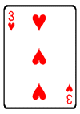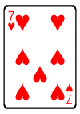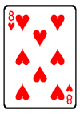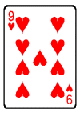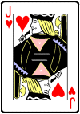This pile has total value 3+4+7+8+9+11=42. A move replaces one of these cards with a lowever vlued one not in the pile. So for example, replacing 8 with 5 or 1 or 2 or the queen are all valid moves. A winning move from this situation is for example replacing 8 by the queen (value 0) decreasing the value from 42 to 34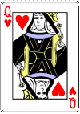But there are otthers, such as replacing 11 by 5, 9 by 1 or 4 by 2. To win this game you need to know the secrets of the tetracode and the MINIMOG.

The tetracode is a one-error correcting code consisting of the following nine words of length four over $\mathbb{F}_3 = { 0,+,- }$

$~\begin{matrix} 0~0 0 0 & 0~+ + + & 0~- – – \\ +~0 + – & +~+ – 0 & +~- 0 + \\ -~0 – + & -~+ 0 – & -~- + 0 \end{matrix}$

The first element (which is slightly offset from the rest) is the slope s of the words, and the other three digits cyclically increase by s (in the field $\mathbb{F}_3$). Because the Hamming-distance is 3 (the minimal number of different digits between two codewords), the tetracode can correct one error, meaning that if at most one of the four digits gets distorted by the channel one can detect and correct this. For example, if you would receive the word $+~++-$ (which is not a codeword) and if you would know that at most one digits went wrong, you can deduce that the word $+~0+-$ was sent. Thus, one can solve the 4-problem for the tetracode : correctt a tetracodeword given all 4 of its digits, one of which may be mistaken.

Another easy puzzle is the 2-problem for the tertracode : complete a tetracodeword from any 2 of its digits. For example, given the incomplete word $?~?0+$ you can decide that the slope should be + and hence that the complete word must be $+~-0+$.

We will use the MINIMOG here as a way to record the blackjack-position. It is a $4 \times 3$ array where the 12 boxes correspond to the card-values by the following scheme

$\begin{array}{|c|ccc|} \hline 6 & 3 & 0 & 9 \\ 5 & 2 & 7 & 10 \\ 4 & 1 & 8 & 11 \\ \hline \end{array}$

and given a blackjack-position we place a star in the corresponding box, so the above start-position (resp. after the first move) corresponds to

$~\begin{array}{|c|ccc|} \hline & \ast & & \ast \\ & & \ast & \\ \ast & & \ast & \ast \\ \hline – & 0 & 0 & + \end{array}~$ respectively $\begin{array}{|c|ccc|} \hline & \ast & \ast & \ast \\ & & \ast & \\ \ast & & & \ast \\ \hline – & 0 & – & + \end{array}$

In the final row we have added elements of $\mathbb{F}_3$ indicating wher ethe stars are placed in that column (if there is just one star, we write the row-number of the star (ordered 0,+,- from top to bottom), if there are two stars we record the row-number of the empty spot. If we would have three or no stars in a column we would record a wild-card character : ?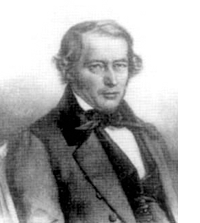Observe that the final row of the start position is $-~00+$ which is NOT a tetracodeword, whereas that of the winning position $-~0-+$ IS a tetracodeword! This is the essence of the _Conway-Ryba winning strategy_ for Mathieu’s blackjack. There are precisely 132 winning positions forming the Steiner-system S(5,6,12). By an S(5,6,12) we mean a collection of 6-element subsets (our card-piles) from a 12-element set (the deck minus the king) having the amazing property that for EVERY 5-tuple from the 12-set there is a UNIQUE 6-element set containing this 5-tuple. Hence, there are exactly $\begin{pmatrix} 12 \\\ 5 \end{pmatrix}/6 = 132$ elements in a Steiner S(5,6,12) system. The winning positions are exactly those MINOMOGs having 6 stars such that the final row is a tetracodeword (or can be extended to a tetracodeword replacing the wildcards ? by suitable digits) and such that the distribution of the stars over the columns is NOT (3,2,1,0) in any order.

Provided the given blackjack-position is not in this Steiner-system (and there is only a 1/7 chance that it is), the strategy is clear : remove one of the stars to get a 5-tuple and determine the unique 6-set of the Steiner-system containing this 5-tuple. If the required extra star corresponds to a value less than the removed star you have a legal and winning move (if not, repeat this for another star). Finding these winning positions means solving 2- and 4-problems for the tetracode. _Another time_ we will say more about this Steiner system and indicate the relation with the Mathieu group $M_{12}$.

References

J.H. Conway and N.J.A. Sloane, ‘The Golay codes and the Mathieu groups’, chp. 10 of “Sphere Packings, Lattices and Groups

David Joyner and Ann Casey-Luers, ‘Kittens, S(5,6,12) and Mathematical blackjack in SAGE

Recently, I’ve been playing with the idea of writing a book for the general public. Its title is still unclear to me (though an idea might be “The disposable science”, better suggestions are of course wellcome) but I’ve fixed the subtitle as “Mathematics’ puzzling fall from grace”. The book’s concept is simple : I would consider the mathematical puzzles creating an hype over the last three centuries : the 14-15 puzzle for the 19th century, Rubik’s cube for the 20th century and, of course, Sudoku for the present century.

For each puzzle, I would describe its origin, the mathematics involved and how it can be used to solve the puzzle and, finally, what the differing quality of these puzzles tells us about mathematics’ changing standing in society over the period. Needless to say, the subtitle already gives away my point of view. The final part of the book would then be more optimistic. What kind of puzzles should we promote for mathematical thinking to have a fighting chance to survive in the near future?